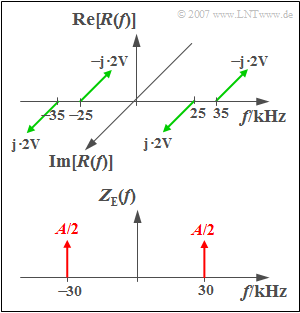# Exercise 3.7: Synchronous DemodulatorThe spectral functions  $R(f)$  and  $Z_{\rm E}(f)$

To reset an amplitude-modulated signal to the original frequency range, a  "Synchronous Demodulator"  is often used:

• This multiplies the AM input signal  $r(t)$  with a carrier signal  $z_{\rm E}(t)$  on the receiver side, which should match the carrier signal  $z_{\rm S}(t)$  on the transmitter side with regard to both frequency  $f_{\rm T}$  and phase  $\varphi_{\rm T}$.
• This is followed by a rectangular low-pass filter to eliminate all spectral components above the carrier frequency  $f_{\rm T}$.  We call the output signal of the synchronous demodulator  $v(t)$.

The spectrum  $R(f)$  of the received signal  $r(t)$  sketched above is produced by  "Two-sideband Amplitude Modulation"  of a sinusoidal source signal  $q(t)$  with the frequency  $5\,\text{kHz}$  and the amplitude  $8\,\text{V}$.  A cosine signal  $z_{\rm S}(t)$  with the frequency  $30\,\text{kHz}$  was used as the transmission-side carrier signal.

The spectrum of the carrier signal  $z_{\rm E}(t)$  on the receiver side consists of two Dirac lines according to the sketch below, each with the weight  $A/2$.  Since  $z_{\rm E}(t)$  is not to contain a unit, the weights of the Dirac functions are also dimensionless.

Hints:

### Questions

1

Let $f_{\rm T} = 30\,\text{kHz}$  and  $A=1$.  Calculate the output signal  $v(t)$.
What signal value occurs at time  $t = 50\, {\rm µ} \text{s}$?

 $v(t = 50\, µ\text{s})\ = \$  $\text{V}$

2

How large must the amplitude of the carrier signal  $z_{\rm E}(t)$  on the receiver side be chosen so that  $v(t) = q(t)$  is valid?

 $A\ = \$

3

Calculate the output signal  $v(t)$  under the conditions  $A = 2$  and $f_{\rm T} = 31\,\text{kHz}$.
What signal value occurs at time  $t = 50\, µ\text{s}$?

 $v(t = 50\, µ\text{s})\ = \$  $\text{V}$

### Solution

#### Solution

(1)  If we name the signal after the multiplier with  $m(t) = r(t) \cdot z_{\rm E}(t)$, the corresponding spectrum  $M(f)$  is the convolution product of  $R(f)$  and  $Z_{\rm E}(f)$.

• Convolution of the spectrum  $R(f)$  with the right Dirac line at  $+30 \text{ kHz}$  leads to discrete spectral lines at  $-\hspace{-0.08cm}5\, \text{kHz}$,  $+5 \,\text{kHz}$,  $+55 \,\text{kHz}$  and  $+65 \,\text{kHz}$.  These are all imaginary and smaller than the impulse weights of  $R(f)$  by a factor of  $A/2 = 0.5$.
• Convolution of  $R(f)$  with the Dirac at  $-\hspace{-0.08cm}30 \,\text{kHz}$  yields lines at  $-\hspace{-0.08cm}65 \,\text{kHz}$,  $-55 \,\text{kHz}$, $-5 \,\text{kHz}$  and  $+5 \,\text{kHz}$.

By superimposing the two intermediate results and taking into account the low-pass filter, which suppresses the lines at  $\pm 55 \text{ kHz}$  and  $\pm 65 \text{ kHz}$ , it thus follows for the spectrum of the sink signal:

$$V( f) = - {\rm{j}} \cdot 2\;{\rm{V}} \cdot \delta ( {f - f_{\rm N} }) + {\rm{j}} \cdot 2\;{\rm{V}} \cdot \delta ( {f + f_{\rm N} } )\hspace{0.3cm}{\rm with}\hspace{0.3cm}f_{\rm N} = 5\;{\rm kHz}.$$
• The sink signal  $v(t)$  is therefore a  $5 \text{ kHz}$–sine signal with amplitude  $4 \text{ V}$.
• The time  $t = 50\, µ\text{s}$  corresponds to a quarter of the period  $T_0 = 1/f_{\rm N} = 200\, µ\text{s}$.
• Thus the sink signal is maximum here, i.e.  $\underline{4 \text{ V}}$.

(2)  With  $A = 1$  the sink signal   $v(t)$  is only half as large as  $q(t)$   ⇒   With  $\underline{A = 2}$  both signals would be equal.

(3)  The Dirac lines at  $\pm f_{\rm T}$  each have weight  $1$.  All spectral lines mentioned below are imaginary and equal in magnitude to  $2 \text{ V}$.

• The convolution of  $R(f)$  with the right Diracl ine of  $z_{\rm E}(t)$  yields components at  $-\hspace{-0.08cm}4\, \text{kHz (p: positive)}$,  $+6 \,\text{kHz (n: negative)}$, $+56 \,\text{kHz (p)}$  and  $+66 \,\text{kHz (n)}$.
• In contrast, the convolution with the left Dirac function leads to spectral lines at  $-\hspace{-0.08cm}66 \,\text{kHz (p)}$,  $-\hspace{-0.08cm}56 \,\text{kHz (n)}$,  $-\hspace{-0.08cm}6 \,\text{kHz (p)}$  und  $+4 \,\text{kHz (n)}$, all also with the (magnitude-related) impulse weights  $2 \text{ V}$.
• Taking the low–pass into account, there are only the four spectral lines at  $\pm 4 \,\text{kHz}$  and  $\pm 6 \,\text{kHz}$.
• The associated time signal is thus with  $f_4 = 4 \,\text{kHz}$  and  $f_6 = 6 \,\text{kHz}$:
$$v( t ) = 4\;{\rm{V}} \cdot \sin ( {2{\rm{\pi }}f_4 t} ) + 4\;{\rm{V}} \cdot \sin ( {2{\rm{\pi }}f_6 t} ) \ne q( t ) = 8\;{\rm{V}} \cdot \sin ( {2{\rm{\pi }}f_5 t} ).$$
• At time  $t = 50\, µ\text{s}$  one obtains:
$$v( t = 50\, µ\text{s}) = 4\;{\rm{V}} \cdot \big[ {\sin \big ( {0.4{\rm{\pi }}} ) + \sin ( {0.6{\rm{\pi }}} )} \big]\hspace{0.15 cm}\underline{ = 7.608\;{\rm{V}}}{\rm{.}}$$